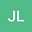•The hypersingular integral equation has been studied widely in boundary element methods, especially in natural boundary element methods. The asymptotic expansion of error function of composite rectangle rule for the computation of Hadamard finite-part integrals with the hypersingular kernel $1/\sin^{2}(x-s)$ is obtained. Extrapolation algorithm is constructed. In order to solve the hypersingular integral equation, the superconvergence point is taken as the collocation point, then the extrapolation algorithm is presented and the convergence rate of extrapolation algorithm for hypersingular integral equation is presented. At last, some numerical results are also illustrated to confirm the theoretical results and show the efficiency of the algorithms.